# 1 Purpose

The purpose of this vignette is to assess the correctness of the functions of the OwenQ package.

As said in the main vignette, the fourth Owen cumulative function $$O_4$$, implemented under the name powen4 allows to evaluate the power of equivalence tests. This is perhaps the main application of the OwenQ package and we will particularly focus on this situation.

Our strategy runs as follows:

• To test the OwenT function, we will compare some values it returns to those returned by other implementations. Note that the other functions of the OwenQ package rely on OwenT when the number of degrees of freedom is odd. Therefore, by testing these other functions for an odd number of degrees of freedom, we implicitely test the OwenT function.

• We will compare a couple of values returned by OwenQ1 and OwenQ2 to the values obtained by numerical integration in Wolfram|Alpha.

• We will test powen4 by performing some power calculations relying on this function and comparing the results with the ones provided by SAS. We will also perform these power calculations with the help of the ipowen4 function, which evaluates the $$O_4$$ function by a powerful numerical integration, and we will compare the results.

• These power calculations will be performed for $$100$$ different scenarios, and we will conclude that powen4 is reliable for these scenarios. Then, to test the other functions of the OwenQ package, we will check that some mathematical relations hold for these $$100$$ scenarios.

The folder tests/testthat of the OwenQ package contains many comparisons with Wolfram|Alpha. The results we obtain with OwenQ are always the same as the ones obtained with Wolfram|Alpha up to a tolerance of at least $$10$$ decimal digits.

# 2 Owen $$T$$-function (OwenT)

The Owen $$T$$-function is implemented under the name OwenT. This is a port of the function owens_t of the C++ special_functions library included in the boost set of libraries. Some details about the C++ implementation are available here.

The libraries provided by boost are peer-reviewed, and this is enough to trust the reliability of OwenT. Nevertheless, below we compare the results of OwenT to the six values given in Patefield’s article, up to $$14$$ decimal digits. We have also checked that Wolfram|Alpha gives these values.

testData <- data.frame(
h = c(0.0625, 6.5, 7, 4.78125, 2, 1),
a = c(0.25, 0.4375, 0.96875, 0.0625, 0.5, 0.9999975),
Patefield = c(3.8911930234701e-02, 2.0005773048508e-11, 6.3990627193899e-13, 1.0632974804687e-07, 8.6250779855215e-03, 6.6741808978229e-02),
OwenT = numeric(6)
)
for(i in 1:nrow(testData)){
testData$OwenT[i] <- OwenT(testData$h[i], testData$a[i]) } print(testData, digits=14) ## h a Patefield OwenT ## 1 0.06250 0.2500000 3.8911930234701e-02 3.8911930234701e-02 ## 2 6.50000 0.4375000 2.0005773048508e-11 2.0005773048508e-11 ## 3 7.00000 0.9687500 6.3990627193899e-13 6.3990627193899e-13 ## 4 4.78125 0.0625000 1.0632974804687e-07 1.0632974804687e-07 ## 5 2.00000 0.5000000 8.6250779855215e-03 8.6250779855215e-03 ## 6 1.00000 0.9999975 6.6741808978229e-02 6.6741808978229e-02 We get the same results with OwenT. # 3 Owen $$Q$$-functions: comparing a couple of values The two Owen $$Q$$-functions $$Q_1$$ and $$Q_2$$ are defined by: $Q_1(\nu, t, \delta, R) = \frac{1}{\Gamma\left(\frac{\nu}{2}\right)2^{\frac12(\nu-2)}} \int_0^R \Phi\left(\frac{tx}{\sqrt{\nu}}-\delta\right) x^{\nu-1} e^{-\frac{x^2}{2}} \mathrm{d}x$ and $Q_2(\nu, t, \delta, R) = \frac{1}{\Gamma\left(\frac{\nu}{2}\right)2^{\frac12(\nu-2)}} \int_R^\infty \Phi\left(\frac{tx}{\sqrt{\nu}}-\delta\right) x^{\nu-1} e^{-\frac{x^2}{2}} \mathrm{d}x.$ They are implemented in the OwenQ package under the respective names OwenQ1 and OwenQ2, for integer values of $$\nu$$, following Owen’s algorithm (1965). In Wolfram|Alpha, these functions are not available, but we can evaluate them by numerical integration. Below we compare two values of OwenQ1 to the ones returned by Wolfram|Alpha. # wolfram: Integrate[(1+Erf[(3*x/Sqrt-2)/Sqrt])*x^(3-1)*Exp[-x^2/2],{x,0,5}]/2/Gamma[3/2]/2^((3-2)/2) OwenQ1(3, 3, 2, 5) ##  0.6800117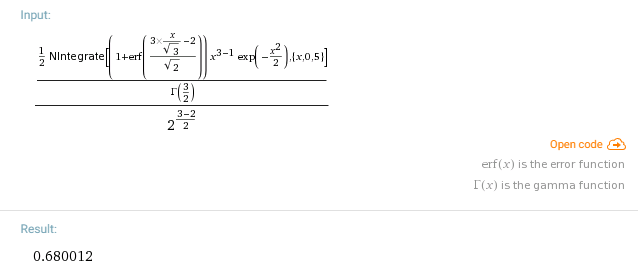Our value rounded to $$6$$ digits is the same as the one given by Wolfram. # wolfram: Integrate[(1+Erf[(3*x/Sqrt-2)/Sqrt])*x^(1000-1)*Exp[-x^2/2],{x,0,30}]/2/Gamma[1000/2]/2^((1000-2)/2) print(OwenQ1(1000, 3, 2, 30), digits=16) ##  0.008518809463589428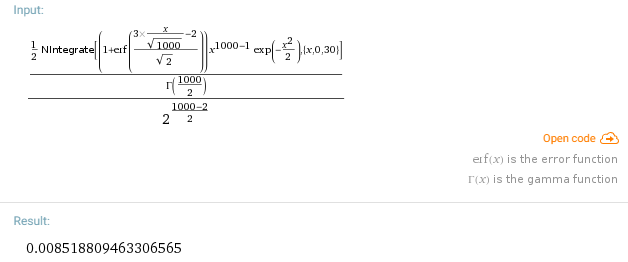The two values are the same up to $$13$$ digits. Now we compare two values of OwenQ2 to the ones returned by Wolfram|Alpha. # wolfram: Integrate[(1+Erf[(3*x/Sqrt-2)/Sqrt])*x^(3-1)*Exp[-x^2/2],{x,5,Infinity}]/2/Gamma[3/2]/2^((3-2)/2) OwenQ2(3, 3, 2, 5) ##  1.54405e-05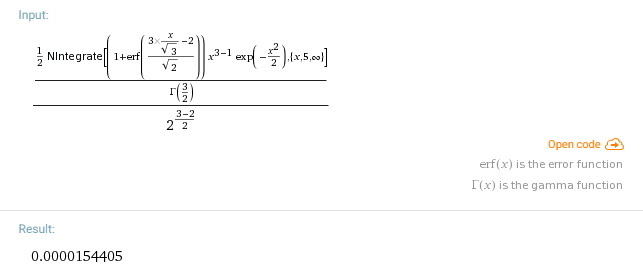The two values are identical. # wolfram: Integrate[(1+Erf[(3*x/Sqrt-2)/Sqrt])*x^(1000-1)*Exp[-x^2/2],{x,5,Infinity}]/2/Gamma[1000/2]/2^((1000-2)/2) print(OwenQ2(1000, 3, 2, 5), digits=16) ##  0.8406201459600969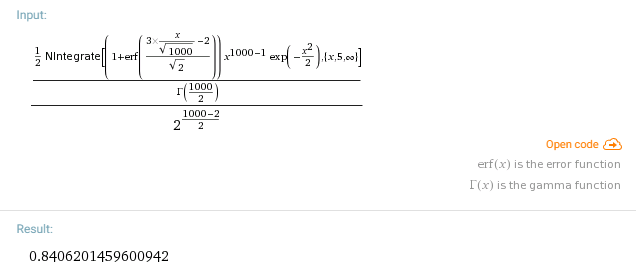The two values are identical up to $$13$$ digits. # 4 Fourth Owen cumulative function $$O_4$$ (powen4) As seen in the other vignette, the fourth Owen cumulative function $$O_4$$, implemented under the name powen4, can be used to evaluate the power of equivalence tests. The powerTOST function below returns the power of the equivalence test for a so-called parallel design with equal variances, when considering the alternative hypothesis $$H_1\colon\{-\Delta < \delta_0 < \Delta \}$$, where $$\delta_0$$ denotes the difference between the two means. This function takes as arguments the significance level $$\alpha$$, the difference $$\delta_0$$ between the two means, the threshold $$\Delta$$, the common standard deviation $$\sigma$$ of the two samples, and the two sample sizes $$n_1$$ and $$n_2$$. powerTOST <- function(alpha, delta0, Delta, sigma, n1, n2, algo=2) { se <- sqrt(1/n1 + 1/n2) * sigma delta1 <- (delta0 + Delta) / se delta2 <- (delta0 - Delta) / se dof <- n1 + n2 - 2 q <- qt(1 - alpha, dof) powen4(dof, q, -q, delta1, delta2, algo=algo) } As you can see, the powen4 function has an argument algo. This is also the case for the other Owen functions, except for ptOwen. The algo argument can take two values, 1 or 2. Th default value is algo=2, and as we will see later, the evaluation is more reliable with this option. The value of algo corresponds to a small difference in the algorithm. With algo=1, the evaluation is a bit faster. And we will see that powerTOST is reliable for algo=1 when the value of n1+n2 is not too large. ## 4.1 Comparisons with SAS The table shown below contains $$100$$ results of power calculations with SAS version 9.4, for a total sample size $$n_1+n_2$$ going from $$20$$ to $$1200$$. The results are rounded to $$5$$ decimal digits. alpha delta0 Delta sigma n1 n2 powerSAS 1 0.05 0.0 1 1 10 10 0.39094 2 0.05 0.0 1 1 15 15 0.69541 3 0.05 0.0 1 1 20 20 0.85580 4 0.05 0.0 1 1 25 25 0.93426 5 0.05 0.0 1 1 30 30 0.97092 6 0.05 0.1 1 1 10 10 0.38272 7 0.05 0.1 1 1 15 15 0.67836 8 0.05 0.1 1 1 20 20 0.83661 9 0.05 0.1 1 1 25 25 0.91781 10 0.05 0.1 1 1 30 30 0.95889 11 0.05 0.2 1 1 10 10 0.35908 12 0.05 0.2 1 1 15 15 0.62954 13 0.05 0.2 1 1 20 20 0.78069 14 0.05 0.2 1 1 25 25 0.86796 15 0.05 0.2 1 1 30 30 0.92017 16 0.05 0.3 1 1 10 10 0.32287 17 0.05 0.3 1 1 15 15 0.55540 18 0.05 0.3 1 1 20 20 0.69322 19 0.05 0.3 1 1 25 25 0.78471 20 0.05 0.3 1 1 30 30 0.84909 21 0.05 0.4 1 1 10 10 0.27820 22 0.05 0.4 1 1 15 15 0.46531 23 0.05 0.4 1 1 20 20 0.58306 24 0.05 0.4 1 1 25 25 0.67174 25 0.05 0.4 1 1 30 30 0.74260 26 0.05 0.5 1 1 10 10 0.22970 27 0.05 0.5 1 1 15 15 0.36967 28 0.05 0.5 1 1 20 20 0.46208 29 0.05 0.5 1 1 25 25 0.53883 30 0.05 0.5 1 1 30 30 0.60600 31 0.05 0.0 1 1 10 30 0.70373 32 0.05 0.0 1 1 15 30 0.85764 33 0.05 0.0 1 1 20 30 0.92324 34 0.05 0.0 1 1 25 30 0.95452 35 0.05 0.0 1 1 30 30 0.97092 36 0.05 0.1 1 1 10 30 0.68648 37 0.05 0.1 1 1 15 30 0.83846 38 0.05 0.1 1 1 20 30 0.90604 39 0.05 0.1 1 1 25 30 0.94003 40 0.05 0.1 1 1 30 30 0.95889 41 0.05 0.2 1 1 10 30 0.63703 42 0.05 0.2 1 1 15 30 0.78255 43 0.05 0.2 1 1 20 30 0.85439 44 0.05 0.2 1 1 25 30 0.89501 45 0.05 0.2 1 1 30 30 0.92017 46 0.05 0.3 1 1 10 30 0.56192 47 0.05 0.3 1 1 15 30 0.69503 48 0.05 0.3 1 1 20 30 0.76944 49 0.05 0.3 1 1 25 30 0.81676 50 0.05 0.3 1 1 30 30 0.84909 51 0.05 0.4 1 1 10 30 0.47061 52 0.05 0.4 1 1 15 30 0.58470 53 0.05 0.4 1 1 20 30 0.65610 54 0.05 0.4 1 1 25 30 0.70594 55 0.05 0.4 1 1 30 30 0.74260 56 0.05 0.5 1 1 10 30 0.37365 57 0.05 0.5 1 1 15 30 0.46343 58 0.05 0.5 1 1 20 30 0.52475 59 0.05 0.5 1 1 25 30 0.57052 60 0.05 0.5 1 1 30 30 0.60600 61 0.05 0.0 3 4 50 50 0.96239 62 0.05 1.0 3 4 50 50 0.79849 63 0.05 2.0 3 4 50 50 0.34329 64 0.05 2.5 3 4 50 50 0.15288 65 0.05 0.0 4 4 10 90 0.81791 66 0.05 1.0 4 4 10 90 0.70346 67 0.05 2.0 4 4 10 90 0.43595 68 0.05 2.5 4 4 10 90 0.29819 69 0.05 0.0 4 7 100 100 0.98278 70 0.05 1.0 4 7 100 100 0.91512 71 0.05 2.0 4 7 100 100 0.64376 72 0.05 3.0 4 7 100 100 0.26169 73 0.01 0.0 4 7 100 100 0.90831 74 0.01 1.0 4 7 100 100 0.74924 75 0.01 2.0 4 7 100 100 0.37443 76 0.01 3.0 4 7 100 100 0.09290 77 0.05 0.0 4 4 185 10 0.84568 78 0.05 1.0 4 4 185 10 0.73025 79 0.05 2.0 4 4 185 10 0.45459 80 0.05 3.0 4 4 185 10 0.19001 81 0.05 0.0 4 8 185 100 0.98240 82 0.05 1.0 4 8 185 100 0.91417 83 0.05 2.0 4 8 185 100 0.64226 84 0.05 3.0 4 8 185 100 0.26103 85 0.01 0.0 4 10 250 250 0.96713 86 0.01 1.0 4 10 250 250 0.84523 87 0.01 2.0 4 10 250 250 0.46161 88 0.01 3.0 4 10 250 250 0.11288 89 0.01 0.0 4 14 500 500 0.97112 90 0.01 1.0 4 14 500 500 0.85433 91 0.01 2.0 4 14 500 500 0.47184 92 0.01 3.0 4 14 500 500 0.11536 93 0.01 0.0 5 35 600 600 0.11547 94 0.01 4.0 5 35 600 600 0.01658 95 0.05 0.0 5 30 600 600 0.78512 96 0.05 4.0 5 50 600 600 0.02642 97 0.01 0.0 5 6 1190 10 0.23194 98 0.01 4.0 5 6 1190 10 0.02739 99 0.05 0.0 5 9 1190 10 0.08256 100 0.05 4.0 5 9 1190 10 0.03115 This table is stored in a dataframe named SAS. We compare the SAS values to the ones obtained by our powerTOST function. power <- numeric(nrow(SAS)) for (i in 1:nrow(SAS)) { power[i] <- powerTOST( alpha = SAS$alpha[i],
delta0 = SAS$delta0[i], Delta = SAS$Delta[i],
sigma = SAS$sigma[i], n1 = SAS$n1[i],
n2 = SAS$n2[i] ) } Rounding our values to $$5$$ decimal digits, we find that our results are identical to the SAS results: identical(round(power,5), SAS$powerSAS)
##  TRUE

With the option algo=1, the results are identical as well:

power <- numeric(nrow(SAS))
for (i in 1:nrow(SAS)) {
power[i] <-
powerTOST(
alpha = SAS$alpha[i], delta0 = SAS$delta0[i],
Delta = SAS$Delta[i], sigma = SAS$sigma[i],
n1 = SAS$n1[i], n2 = SAS$n2[i],
algo = 1
)
}
identical(round(power,5), SAS$powerSAS) ##  TRUE ## 4.2 Comparison with numerical integration The ipowen4 internal function of the OwenQ package evaluates the fourth Owen cumulative function $$O_4$$ by a powerful numerical integration implemented in C++ (with the package RcppNumerical). Thus we have two completely different implementations of $$O_4$$. The ipowerTOST function below is obtained by replacing powen4 with ipowen4 in powerTOST. We will compare the results of powerTOST with the ones of ipowerTOST. ipowerTOST <- function(alpha, delta0, Delta, sigma, n1, n2) { se <- sqrt(1/n1 + 1/n2) * sigma delta1 <- (delta0 + Delta) / se delta2 <- (delta0 - Delta) / se dof <- n1 + n2 - 2 q <- qt(1 - alpha, dof) OwenQ:::ipowen4(dof, q, -q, delta1, delta2) } ### 4.2.1 Failures and successes of powen4 • For $$n_1=n_2=1000$$, $$\alpha=0.05$$, $$\delta=0$$, $$\Delta=5$$, the powen4 function with the option algo=1 abnormally takes some negative values: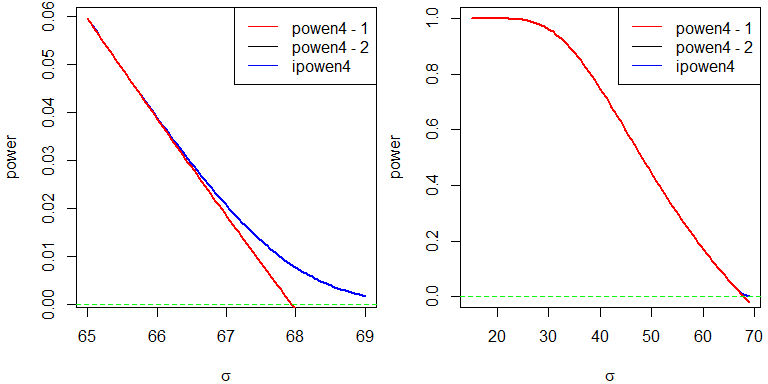Note that the discrepancy between powen4 and ipowen4 occurs only for $$\sigma > 65$$. • For $$n_1=n_2=720$$, $$\alpha=0.05$$, $$\delta=0$$, $$\Delta=5$$, we observe a discrepancy between powen4 with the option algo=1 and ipowen4: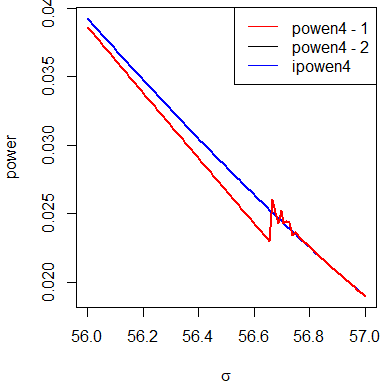There is no discrepancy between powen4 with the option algo=2 and ipowen4. The irregularity of powen4 with the option algo=1 suggests that it returns wrong values. • For $$n_1=n_2=700$$, $$\alpha=0.01$$, $$\delta=0$$, $$\Delta=5$$, we still observe a small discrepancy between powen4 with the option algo=1 and ipowen4, and we still observe an irregularity of powen4 (on the second figure below):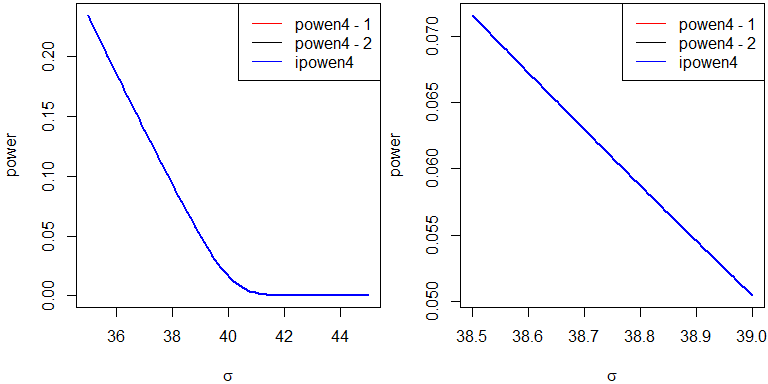With the option algo=2, the results of powen4 coincide with the ones of ipowen4. • For $$n_1 = n_2 = 600$$, $$\alpha=0.005$$, $$\delta=0$$, $$\Delta=5$$, we do not observe any discrepancy between powen4 and ipowen4: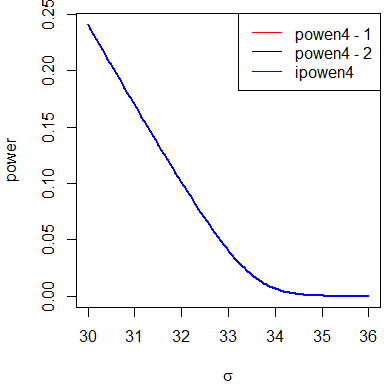We conclude that powen4 with the option algo=1 is not reliable when the number of degrees of freedom is too large. As said before, the interest of the option algo=1 is that the evaluation is faster. For $$n_1=n_2=2500$$, the results of powerTOST with algo=2 still coincide with the result of ipowerTOST: alpha <- 0.05; delta0 <- 0; Delta <- 5 sigma <- 110 n1 <- n2 <- 2500 powerTOST(alpha, delta0, Delta, sigma, n1, n2) ##  4.523596e-05 ipowerTOST(alpha, delta0, Delta, sigma, n1, n2) ##  4.523596e-05 ## attr(,"err_est") ##  5.022201e-19 ## attr(,"err_code") ##  0 And even for $$n_1=n_2=5000$$: sigma <- 152 n1 <- n2 <- 5000 powerTOST(alpha, delta0, Delta, sigma, n1, n2) ##  0.003612374 ipowerTOST(alpha, delta0, Delta, sigma, n1, n2) ##  0.003612374 ## attr(,"err_est") ##  4.010541e-17 ## attr(,"err_code") ##  0 ### 4.2.2 Comparisons for $$n_1+n_2 \leq 1200$$ Below we compare the results returned by powerTOST to the ones returned by ipowerTOST for the parameters given in the SAS dataset. power <- ipower <- numeric(nrow(SAS)) for (i in 1:nrow(SAS)) { power[i] <- powerTOST( alpha = SAS$alpha[i],
delta0 = SAS$delta0[i], Delta = SAS$Delta[i],
sigma = SAS$sigma[i], n1 = SAS$n1[i],
n2 = SAS$n2[i] ) ipower[i] <- ipowerTOST( alpha = SAS$alpha[i],
delta0 = SAS$delta0[i], Delta = SAS$Delta[i],
sigma = SAS$sigma[i], n1 = SAS$n1[i],
n2 = SAS$n2[i] ) } identical(round(power, 10), round(ipower, 10)) ##  TRUE Results are the same up to $$10$$ digits. And also with the option algo=1: power <- numeric(nrow(SAS)) for (i in 1:nrow(SAS)) { power[i] <- powerTOST( alpha = SAS$alpha[i],
delta0 = SAS$delta0[i], Delta = SAS$Delta[i],
sigma = SAS$sigma[i], n1 = SAS$n1[i],
n2 = SAS$n2[i], algo = 1 ) } identical(round(power, 10), round(ipower, 10)) ##  TRUE ## 4.3 Conclusion • The powen4 function with option algo=1 seems to return correct values for $$\nu \leq 1200$$. • The powen4 function with option algo=2 allows higher values of $$\nu$$. • In any case, we recommend to compare the result of powen4 with the one of ipowen4. # 5 Other Owen cumulative functions We previously validated the powen4 function for the $$100$$ scenarios of the dataset SAS. Now we test the functions powen1, powen2 and powen3 by checking the equality $O_1(\nu, t_1, t_2, \delta_1, \delta_2) + O_2(\nu, t_1, t_2, \delta_1, \delta_2) + O_3(\nu, t_1, t_2, \delta_1, \delta_2) + O_4(\nu, t_1, t_2, \delta_1, \delta_2) = 1.$ We restrict our attention to default setting algo=2. We check the above equality for the $$100$$ scenarios of the dataset SAS. f <- function(alpha, delta0, Delta, sigma, n1, n2) { se <- sqrt(1/n1 + 1/n2) * sigma delta1 <- (delta0 + Delta) / se delta2 <- (delta0 - Delta) / se dof <- n1 + n2 - 2 q <- qt(1 - alpha, dof) powen1(dof, q,-q, delta1, delta2) + powen2(dof, q,-q, delta1, delta2) + powen3(dof, q,-q, delta1, delta2) + powen4(dof, q,-q, delta1, delta2) } test <- numeric(nrow(SAS)) for (i in 1:nrow(SAS)) { test[i] <- f( alpha = SAS$alpha[i],
delta0 = SAS$delta0[i], Delta = SAS$Delta[i],
sigma = SAS$sigma[i], n1 = SAS$n1[i],
n2 = SAS$n2[i] ) } all(abs(test-1) < 1e-14) ##  TRUE We find that each of the $$100$$ sums is equal to $$1$$ up to a tolerance of $$14$$ digits. # 6 First Owen $$Q$$-function $$Q_1$$ (OwenQ1) We previously validated the powen4 function (implementation of the fourth Owen cumulative function $$O_4$$) for the $$100$$ scenarios of the dataset SAS. Now, to test OwenQ1, we will use the following equality: $O_4(\nu, t_1, t_2, \delta_1, \delta_2) = Q_1\left(\nu, t_2, \delta_2, \frac{\sqrt{\nu}(\delta_1-\delta_2)}{t_1-t_2}\right) - Q_1\left(\nu, t_1, \delta_1, \frac{\sqrt{\nu}(\delta_1-\delta_2)}{t_1-t_2}\right).$ We use this formula to perform the power calculations with OwenQ1 instead of powen4. powerTOST2 <- function(alpha, delta0, Delta, sigma, n1, n2) { se <- sqrt(1/n1 + 1/n2) * sigma delta1 <- (delta0 + Delta) / se delta2 <- (delta0 - Delta) / se dof <- n1 + n2 - 2 q <- qt(1 - alpha, dof) R <- sqrt(dof)*(delta1 - delta2)/q/2 OwenQ1(dof, -q, delta2, R) - OwenQ1(dof, q, delta1, R) } power2 <- numeric(nrow(SAS)) for (i in 1:nrow(SAS)) { power2[i] <- powerTOST2( alpha = SAS$alpha[i],
delta0 = SAS$delta0[i], Delta = SAS$Delta[i],
sigma = SAS$sigma[i], n1 = SAS$n1[i],
n2 = SAS$n2[i] ) } all(abs(power - power2) < 1e-9) ##  TRUE The values rounded to $$9$$ digits are the same as the ones we previously obtained with powen4. # 7 Second Owen $$Q$$-function $$Q_2$$ (OwenQ2) We previously validated powen2 for the $$100$$ scenarios of the dataset SAS. Now we test the OwenQ2 function by checking the equality $O_2(\nu, t_1, t_2, \delta_1, \delta_2) = Q_2\left(\nu, t_1, \delta_1, \frac{\sqrt{\nu}(\delta_1-\delta_2)}{t_1-t_2}\right) - Q_2\left(\nu, t_2, \delta_2, \frac{\sqrt{\nu}(\delta_1-\delta_2)}{t_1-t_2}\right).$ We check this equality for the $$100$$ scenarios of the dataset SAS. g <- function(alpha, delta0, Delta, sigma, n1, n2) { se <- sqrt(1/n1 + 1/n2) * sigma delta1 <- (delta0 + Delta) / se delta2 <- (delta0 - Delta) / se dof <- n1 + n2 - 2 q <- qt(1 - alpha, dof) R <- sqrt(dof)*(delta1 - delta2)/q/2 x <- OwenQ2(dof, q, delta1, R) - OwenQ2(dof, -q, delta2, R) y <- powen2(dof, q, -q, delta1, delta2) x - y } test <- numeric(nrow(SAS)) for (i in 1:nrow(SAS)) { test[i] <- g( alpha = SAS$alpha[i],
delta0 = SAS$delta0[i], Delta = SAS$Delta[i],
sigma = SAS$sigma[i], n1 = SAS$n1[i],
n2 = SAS$n2[i] ) } all(abs(test) < 1e-15) ##  TRUE We find that each of the $$100$$ equalities hold true up to a tolerance of $$15$$ digits. # 8 Student cumulative distribution function (ptOwen) We finally test ptOwen, the implementation of the cumulative distribution function of the noncentral Student distribution. If $$T_1$$ follows the noncentral Student distribution with number of degrees of freedom $$\nu$$ and noncentrality parameter $$\delta_1$$, then for any $$t_1$$ the equality $\Pr(T_1 \leq t_1) = O_1(\nu, t_1, t_2, \delta_1, \delta_2) + O_2(\nu, t_1, t_2, \delta_1, \delta_2)$ holds for any $$t_2$$ and $$\delta_2$$. We check this equality for the $$100$$ scenarios of the dataset SAS. h <- function(alpha, delta0, Delta, sigma, n1, n2) { se <- sqrt(1/n1 + 1/n2) * sigma delta1 <- (delta0 + Delta) / se delta2 <- (delta0 - Delta) / se dof <- n1 + n2 - 2 q <- qt(1 - alpha, dof) x <- ptOwen(q, dof, delta1) y <- powen1(dof, q, -q, delta1, delta2) + powen2(dof, q, -q, delta1, delta2) x - y } test <- numeric(nrow(SAS)) for (i in 1:nrow(SAS)) { test[i] <- h( alpha = SAS$alpha[i],
delta0 = SAS$delta0[i], Delta = SAS$Delta[i],
sigma = SAS$sigma[i], n1 = SAS$n1[i],
n2 = SAS\$n2[i]
)
}
all(abs(test) < 1e-15)
##  TRUE

For each scenario, the equality holds up to a tolerance of $$15$$ digits.